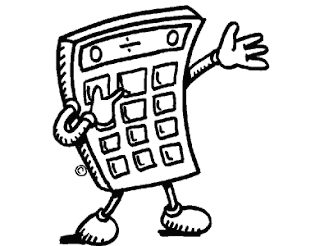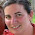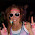## Monday, April 23, 2012

### Calculator Fun!

So, with the math test coming up, I have to make sure that my kids know how to use a calculator.  Since I'm looping, that shouldn't be a problem...I taught them last year....BUT, for those of you who might be teaching calculator skills for the first time, I'm sharing with you the lesson that I do each year.HOOK:  Ask a student for a whole number 1-20.  Have them write it down on a piece of paper or tell a friend.  You may not see the number!  Tell the students that you are a "mathemagician" and you are going to use your math powers to figure out the number they chose.

Have the student type the secret number into their calculator.  Then have them follow these steps:
1.  Multiply by 3
2.  Add the secret number, then subtract 5.
3.  Multiply by 3, then multiply by 3 again.
4.  Add the secret number, then subtract the number of their favorite month (month doesn't matter to you)
5.  Multiply by 3, then multiply by 3 again, and then again a third time.
6.  Add the secret number, then subtract their favorite day of the month (again, doesn't matter to you)
7.  Have them show you what they got.
Here is where you become a magician in their minds:

The number is going to be big.  No worries, it just makes you seem that much smarter!!

If the result is negative, their secret number was 1 (or they cheated or messed up)
If the result has only three digits, their secret number was 2
If neither of those are the case, ignore the last three digits, and add 2!  That's the secret number!  It works, try it!  :)

Then we break into partners.  I then have them do birthday math (2 times-1 for each partner).
I tell them to have partner 1 type in the month and day of their birthday into the calculator.  If the day is a single digit it should be recorded as a two-digit number (example June 7 would be 0607).  I usually have to talk them through that the first time.  Then I call out the following rules:
1.  Multiply the birthday number by 2
3. Multiply this answer by 50
4.  Subtract 250. The first four digits disclose the person’s birthday.
They then switch partners and do the same thing again.  I usually have these steps on the Smartboard and pull down the shade one step at a time on the first rotation, then let them loose on the second.  I  then give them this worksheet and let them work in partners.

They think it's SOOOOO cool! If your kids already know how to use calculators, they will still love this!  I won't lie, I'm always amazed that it works...every year.  Silly, I know. This can be a center too.

9078 on.
(in digital number font, upside down)

1.2.1.